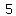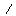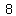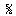U+215D VULGAR FRACTION FIVE EIGHTHS

U+215D was added to Unicode in version 1.1 (1993). It belongs to the blockNumber Forms in the Basic Multilingual Plane.

This character is a Other Number and is commonly used, that is, in no specific script. The codepoint has the Numeric value 5/8.

A fraction (from Latin: fractus, "broken") represents a part of a whole or, more generally, any number of equal parts. When spoken in everyday English, a fraction describes how many parts of a certain size there are, for example, one-half, eight-fifths, three-quarters. A common, vulgar, or simple fraction (examples: and 17/3) consists of an integer numerator, displayed above a line (or before a slash), and a non-zero integer denominator, displayed below (or after) that line. Numerators and denominators are also used in fractions that are not common, including compound fractions, complex fractions, and mixed numerals.

The numerator represents a number of equal parts, and the denominator, which cannot be zero, indicates how many of those parts make up a unit or a whole. For example, in the fraction 3/4, the numerator, 3, tells us that the fraction represents 3 equal parts, and the denominator, 4, tells us that 4 parts make up a whole. The picture to the right illustrates or 3/4 of a cake.

Fractional numbers can also be written without using explicit numerators or denominators, by using decimals, percent signs, or negative exponents (as in 0.01, 1%, and 10−2 respectively, all of which are equivalent to 1/100). An integer such as the number 7 can be thought of as having an implicit denominator of one: 7 equals 7/1.

Other uses for fractions are to represent ratios and to represent division. Thus the fraction 3/4 is also used to represent the ratio 3:4 (the ratio of the part to the whole) and the division 3 ÷ 4 (three divided by four).

In mathematics the set of all numbers which can be expressed in the form a/b, where a and b are integers and b is not zero, is called the set of rational numbers and is represented by the symbol Q, which stands for quotient. The test for a number being a rational number is that it can be written in that form (i.e., as a common fraction). However, the word fraction is also used to describe mathematical expressions that are not rational numbers, for example algebraic fractions (quotients of algebraic expressions), and expressions that contain irrational numbers, such as √2/2 (see square root of 2) and π/4 (see proof that π is irrational).

Representations

System Representation
8541
UTF-8 E2 85 9D
UTF-16 21 5D
UTF-32 00 00 21 5D
URL-Quoted %E2%85%9D
HTML-Escape &#x215D;
Wrong windows-1252 Mojibake â
HTML-Escape &frac58;
digraph 58
LaTeX \textfrac{5}{8}
Encoding: EUC-KR (hex bytes) A8 FD

Complete Record

Property Value
Age (age) 1.1
Unicode Name (na) VULGAR FRACTION FIVE EIGHTHS
Unicode 1 Name (na1) FRACTION FIVE EIGHTHS
Block (blk) Number_Forms
General Category (gc) Other Number
Script (sc) Common
Bidirectional Category (bc) Other Neutral
Combining Class (ccc) Not Reordered
Decomposition Type (dt) Fraction
Decomposition Mapping (dm)Lowercase (Lower)
Simple Lowercase Mapping (slc)Lowercase Mapping (lc)Uppercase (Upper)
Simple Uppercase Mapping (suc)Uppercase Mapping (uc)Simple Titlecase Mapping (stc)Titlecase Mapping (tc)Case Folding (cf)ASCII Hex Digit (AHex)
Alphabetic (Alpha)
Bidi Control (Bidi_C)
Bidi Mirrored (Bidi_M)
Bidi Paired Bracket (bpb)Bidi Paired Bracket Type (bpt) None
Cased (Cased)
Composition Exclusion (CE)
Case Ignorable (CI)
Full Composition Exclusion (Comp_Ex)
Changes When Casefolded (CWCF)
Changes When Casemapped (CWCM)
Changes When NFKC Casefolded (CWKCF)
Changes When Lowercased (CWL)
Changes When Titlecased (CWT)
Changes When Uppercased (CWU)
Dash (Dash)
Deprecated (Dep)
Default Ignorable Code Point (DI)
Diacritic (Dia)
East Asian Width (ea) Ambiguous
Extender (Ext)
FC NFKC Closure (FC_NFKC)Grapheme Cluster Break (GCB) Any
Grapheme Base (Gr_Base)
Grapheme Extend (Gr_Ext)
Hex Digit (Hex)
Hangul Syllable Type (hst) Not Applicable
Hyphen (Hyphen)
ID Continue (IDC)
Ideographic (Ideo)
ID Start (IDS)
IDS Binary Operator (IDSB)
IDS Trinary Operator and (IDST)
InMC (InMC)
Indic Positional Category (InPC) NA
Indic Syllabic Category (InSC) Other
ISO 10646 Comment (isc)
Joining Group (jg) No_Joining_Group
Join Control (Join_C)
Jamo Short Name (JSN)
Joining Type (jt) Non Joining
Line Break (lb) Alphabetic
Logical Order Exception (LOE)
Math (Math)
Noncharacter Code Point (NChar)
NFC Quick Check (NFC_QC) Yes
NFD Quick Check (NFD_QC) Yes
NFKC Casefold (NFKC_CF)NFKC Quick Check (NFKC_QC) No
NFKD Quick Check (NFKD_QC) No
Numeric Type (nt) Numeric
Numeric Value (nv) 5/8
Other Alphabetic (OAlpha)
Other Default Ignorable Code Point (ODI)
Other Grapheme Extend (OGr_Ext)
Other ID Continue (OIDC)
Other ID Start (OIDS)
Other Lowercase (OLower)
Other Math (OMath)
Other Uppercase (OUpper)
Pattern Syntax (Pat_Syn)
Pattern White Space (Pat_WS)
Quotation Mark (QMark)
Sentence Break (SB) Other
Simple Case Folding (scf)Script Extension (scx) Common
Soft Dotted (SD)
STerm (STerm)
Terminal Punctuation (Term)
Unified Ideograph (UIdeo)
Variation Selector (VS)
Word Break (WB) Other
White Space (WSpace)
XID Continue (XIDC)
XID Start (XIDS)
Expands On NFC (XO_NFC)
Expands On NFD (XO_NFD)
Expands On NFKC (XO_NFKC)
Expands On NFKD (XO_NFKD)